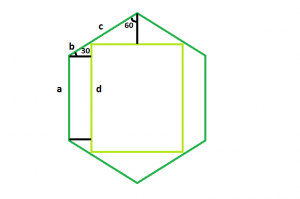# Largest Square that can be inscribed within a hexagon

Given a regular hexagonof side length a, the task is to find the area of the largest square that can be inscribed within it.
Examples:

Input: a = 6
Output: 57.8817
Input: a = 8
Output: 102.901Approach: The square we will derive will have the same centre and axes of the hexagon. This is because the square will become smaller if we will rotate it.

The sides of the hexagonal are equal i.e. a = b + c
Now, let d be the length of the side of the inscribed square,
Then the top side of the square, d = 2 * c * sin(60)
And, the left side of the square, d = a + 2 * b * sin(30)
Substituting for c, d = 2 * (a – b) * sin(60)
Now taking d and re-arranging, we get, b / a = (2 * sin(60) – 1) / (2 * (sin(30) + sin(60)))
So, b / a = 2 – ?3
Now, substituting the relation of b and a in the left hand side equation of square, we get,
d / a = 3 – ?3 i.e. d / a = 1.268
Therefore, d = 1.268 * a

Below is the implementation of the above approach:

## C++

 `// C++ program to find the area of the largest square` `// that can be inscribed within the hexagon` `#include ` `using` `namespace` `std;`   `// Function to find the area` `// of the square` `float` `squareArea(``float` `a)` `{`   `    ``// Side cannot be negative` `    ``if` `(a < 0)` `        ``return` `-1;`   `    ``// Area of the square` `    ``float` `area = ``pow``(1.268, 2) * ``pow``(a, 2);` `    ``return` `area;` `}`   `// Driver code` `int` `main()` `{` `    ``float` `a = 6;` `    ``cout << squareArea(a) << endl;` `    ``return` `0;` `}`

## Java

 `// Java program to find the area of the largest square` `// that can be inscribed within the hexagon` `class` `Solution {` `    ``// Function to find the area` `    ``// of the square` `    ``static` `float` `squareArea(``float` `a)` `    ``{`   `        ``// Side cannot be negative` `        ``if` `(a < ``0``)` `            ``return` `-``1``;`   `        ``// Area of the square` `        ``float` `area = (``float``)(Math.pow(``1.268``, ``2``) * Math.pow(a, ``2``));` `        ``return` `area;` `    ``}`   `    ``// Driver code` `    ``public` `static` `void` `main(String args[])` `    ``{` `        ``float` `a = ``6``;` `        ``System.out.println(squareArea(a));` `    ``}` `}`   `// This code is contributed by Arnab Kundu`

## Python3

 `# Python program to find the area of the largest square` `# that can be inscribed within the hexagon`   `# Function to find the area` `# of the square` `def` `squareArea(a):`   `    ``# Side cannot be negative` `    ``if` `(a < ``0``):` `        ``return` `-``1``;`   `    ``# Area of the square` `    ``area ``=` `(``1.268` `*``*` `2``) ``*` `(a ``*``*` `2``);` `    ``return` `area;`   `# Driver code` `a ``=` `6``;` `print``(squareArea(a));`   `# This code contributed by PrinciRaj1992 `

## C#

 `// C# program to find the area of the largest square` `// that can be inscribed within the hexagon` `using` `System;` `class` `Solution {` `    ``// Function to find the area` `    ``// of the square` `    ``static` `float` `squareArea(``float` `a)` `    ``{`   `        ``// Side cannot be negative` `        ``if` `(a < 0)` `            ``return` `-1;`   `        ``// Area of the square` `        ``float` `area = (``float``)(Math.Pow(1.268, 2) * Math.Pow(a, 2));` `        ``return` `area;` `    ``}`   `    ``// Driver code` `    ``public` `static` `void` `Main()` `    ``{` `        ``float` `a = 6;` `        ``Console.WriteLine(squareArea(a));` `    ``}` `}`   `// This code is contributed by  anuj_67..`

## PHP

 ``

## Javascript

 ``

Output:

`57.8817`

Time Complexity: O(1)

Auxiliary Space: O(1)

Feeling lost in the world of random DSA topics, wasting time without progress? It's time for a change! Join our DSA course, where we'll guide you on an exciting journey to master DSA efficiently and on schedule.
Ready to dive in? Explore our Free Demo Content and join our DSA course, trusted by over 100,000 geeks!

Previous
Next